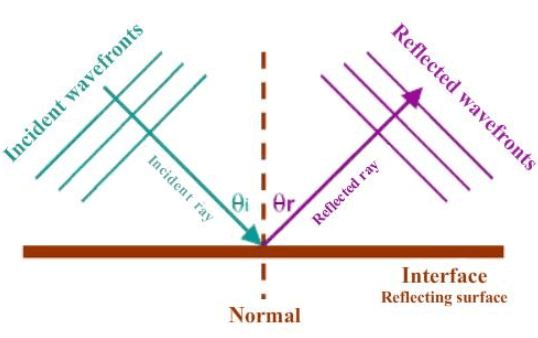Courses

# Theory - To verify the laws of reflections of sound, Physics, Science Class 9 Notes | EduRev

## Class 9 : Theory - To verify the laws of reflections of sound, Physics, Science Class 9 Notes | EduRev

The document Theory - To verify the laws of reflections of sound, Physics, Science Class 9 Notes | EduRev is a part of the Class 9 Course Science Class 9.
All you need of Class 9 at this link: Class 9

Our Objective

To verify the laws of reflections of sound.The Theory

What is reflection?

Reflection is the change in direction of a wavefront at an interface between two different media so that the wavefront returns into the medium from which it originated. Common examples include the reflection of light, sound and water waves.

Do you know how sound propagates?

Sound propagates through air as a longitudinal wave. The speed of sound is determined by the properties of the air, and not by the frequency or amplitude of the sound.  If a sound is not absorbed or transmitted when it strikes a surface, it is reflected. The law for reflection is the same as that of light, ie., the angle of incidence of a sound wave equals the angle of reflection, just as if it were produced by a 'mirror image' of the stimulus on the opposite side of the surface.

How do we describe the reflection of sound?

When sound travels in a given medium, it strikes the surface of another medium and bounces back in some other direction, this phenomenon is called the reflection of sound. The waves are called the incident and reflected sound waves.

What are incident and reflected sound waves?

The sound waves that travel towards the reflecting surface are called the incident sound waves. The sound waves bouncing back from the reflecting surface are called reflected sound waves. For all practical purposes, the point of incidence and the point of reflection are the same point on the reflecting surface.

A perpendicular drawn on the point of incidence is called the normal. The angle which the incident sound waves makes with the normal is called the angle of incidence, "i". The angle which the reflected sound waves makes with the normal is called the angle of reflection, "r".

Let’s look at the two laws of reflection

The following two laws of reflection of light are applicable to sound waves as well:

• The incident wave, the normal to the reflecting surface and the reflected wave at the point of incidence lie in the same plane.

• The angle of incidence ∠i is equal to the angle of reflection ∠r.

Learning Outcomes

Student will understand the First and Second Laws of Reflection.

First Law of ReflectionThe incident wave, the reflected wave, and the normal at the point of incidence lie on the same plane.

Law of ReflectionThe angle of incidence is equal to the angle of reflection.

Offer running on EduRev: Apply code STAYHOME200 to get INR 200 off on our premium plan EduRev Infinity!

## Science Class 9

143 videos|224 docs|135 tests

,

,

,

,

,

,

,

,

,

,

,

,

,

,

,

,

,

,

,

,

,

,

,

,

,

,

,

;